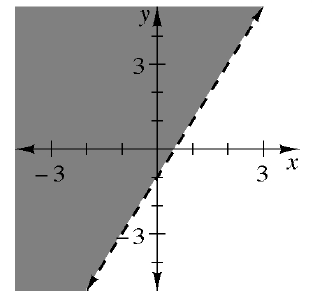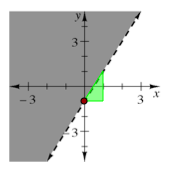### Home > CCA2 > Chapter C > Lesson C.1.1 > ProblemC-7

C-7.

Write the inequality represented by the graph below.Find the equation of the line, $y = mx + b$.
$m =$ slope (growth) and $b = y$-intercept.

Draw a growth triangle and find the $y$-intercept.Is the shaded area above or below the line?
If the shaded area is above the line, the inequality is greater than the line.
If the shaded area is below the line, the inequality is less than the line.

A less than $(<)$ or greater than $(>)$ sign is a dotted line,
while a less than or equal to $(≤)$ or greater than or equal to $(≥)$ sign is a solid line.

$y > 2x - 1$

Use the eTool below to solve the problem.
Click the link at the right to view full version of eTool: CCA2 C-7 HW eTool